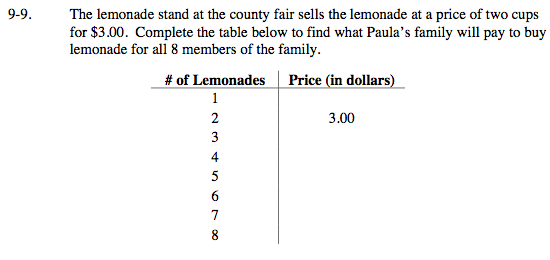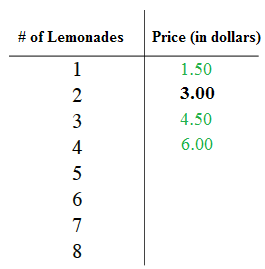### Home > MC2 > Chapter 9 > Lesson 9.1.1 > Problem9-9

9-9.Since 2 cups of lemonade is twice as much as 1 cup of lemonade, to find the price of 1 cup of lemonade, you must half the price of 2 cups of lemonade.

You can also solve the problem by setting a proportion equation like the one below, then solve for x.

$\frac{\text{2 cups of lemonade}}{\text{3 dollars}} \left( \frac{?}{?} \right) = \frac{\text{1 cup of lemonade}}{x\text{ dollars}}$

To find the price of any amount of lemonade, in the equation above substitute ''1 cup of lemonade'' with the number of lemonades you are looking for.